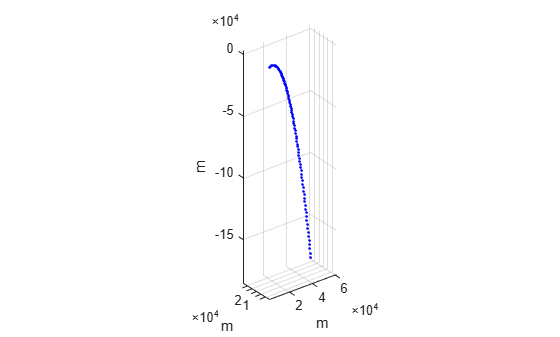Documentation

# step

System object: phased.Platform
Package: phased

Output current position, velocity, and orientation axes of platform

## Syntax

```[Pos,Vel] = step(sPlat,T) [Pos,Vel] = step(sPlat,T,V) [Pos,Vel] = step(sPlat,T,A) [Pos,Vel,Laxes] = step(___) ```

## Description

### Note

Starting in R2016b, instead of using the `step` method to perform the operation defined by the System object™, you can call the object with arguments, as if it were a function. For example, `y = step(obj,x)` and `y = obj(x)` perform equivalent operations.

`[Pos,Vel] = step(sPlat,T)` returns the current position, `Pos`, and velocity, `Vel`, of the platform. The method then updates the position and velocity. When the `MotionModel` property is set to `'Velocity'` and the `VelocitySource` property is set to `'Property'`, the position is updated using the equation Pos = Pos + Vel*T where T specifies the elapsed time (in seconds) for the current step. When the `MotionModel` property is set to `'Acceleration'` and the `AccelerationSource` property is set to `'Property'`, the position and velocity are updated using the equations Pos = Pos + Vel*T + 1/2Acl*T^2 and Vel = Vel + Acl*T where T specifies the elapsed time (in seconds) for the current step.

`[Pos,Vel] = step(sPlat,T,V)` returns the current position, `Pos`, and the current velocity, `Vel`, of the platform. The method then updates the position and velocity using the equation Pos = Pos + Vel*T where T specifies the elapsed time (in seconds) for the current step. This syntax applies when you set the `MotionModel` property to `'Velocity'` and the `VelocitySource` property to ```'Input port'```.

`[Pos,Vel] = step(sPlat,T,A)` returns the current position, `Pos`, and the current velocity, `Vel`, of the platform. The method then updates the position and velocity using the equations Pos = Pos + Vel*T + 1/2Acl*T^2 and Vel = Vel + Acl*T where T specifies the elapsed time (in seconds) for the current step. This syntax applies when you set the `MotionModel` property to `'Acceleration'` and the `AccelerationSource` property to ```'Input port'```.

`[Pos,Vel,Laxes] = step(___)` returns the additional output `Laxes` as the platform's orientation axes when you set the `OrientationAxesOutputPort` property to `true`.

### Note

The object performs an initialization the first time the object is executed. This initialization locks nontunable properties (MATLAB) and input specifications, such as dimensions, complexity, and data type of the input data. If you change a nontunable property or an input specification, the System object issues an error. To change nontunable properties or inputs, you must first call the `release` method to unlock the object.

## Input Arguments

 `sPlat` Platform Platform, specified as a `phased.Platform` System object. `T` Step time Step time, specified as a real-valued scalar. Units are seconds `V` Platform velocity Platform velocity, specified as a real-valued 3-by-N matrix where N is the number of platforms to model. This argument applies when you set the `MotionModel` property to `'Velocity'` and the `VelocitySource` property to `'Input port'`. Units are meters per second. `A` Platform acceleration Platform acceleration, specified as a real-valued 3-by-N matrix where N is the number of platforms to model. This argument applies when you set the `MotionModel` property to `'Acceleration'` and the `AccelerationSource` property to `'Input port'`. Units are meters per second-squared.

## Output Arguments

 `Pos` Current platform position Current position of platform, specified as a real-valued 3-by-1 column vector in the form of `[x;y;z]` or a real-valued 3-by-N matrix where N is the number of platforms to model. Each column takes the form `[x;y;z]`. Units are meters. `Vel` Current platform velocity Current velocity of platform, specify as a real-valued 3-by-1 column vector in the form of `[vx;vy;vz]` or a real-valued 3-by-N matrix where N is the number of platforms to model. Each column taking the form `[vx;vy;vz]`. Velocity units are meters per second. `Laxes` Current platform orientation axes Current platform orientation axes, returned as real-valued 3-by-3-by-N matrix where N is the number of platforms to model. Each 3-by-3 submatrix is an orthonormal matrix. This output is enabled when you set the `OrientationAxesOutputPort` property to `true`. The current platform axes rotate around the normal vector to the path of the platform.

## Examples

expand all

Create two moving platforms. The first platform, starting at the origin, has a velocity of (100,100,0) meters per second. The second starts at (1000,0,0) meters and has a velocity of (0,200,0) meters per second. Next, specify different local coordinate axes for each platform defined by rotation matrices. Setting the `OrientationAxesOutputPort` property to `true` lets you retrieve the local coordinate axes at each step.

Set up the platform object.

```pos0 = [[0;0;0],[1000;0;0]]; vel0 = [[100;100;0],[0;200;0]]; R1 = rotx(30); R2 = roty(45); laxes(:,:,1) = R1; laxes(:,:,2) = R2; sPlat = phased.Platform(pos0,vel0,... 'OrientationAxesOutputPort',true,... 'InitialOrientationAxes',laxes);```

Simulate the motion of the platform for two time steps, assuming the time elapsed for each step is one second. The position of the platform is updated after each step.

`T = 1;`

At the first step, the position and velocity equal the initial values.

```[pos,v,lax] = step(sPlat,T); pos```
```pos = 3×2 0 1000 0 0 0 0 ```
`lax`
```lax = lax(:,:,1) = 1.0000 0 0 0 0.8660 -0.5000 0 0.5000 0.8660 lax(:,:,2) = 0.7071 0 0.7071 0 1.0000 0 -0.7071 0 0.7071 ```

At the second step, the position is updated.

```[pos,v,lax] = step(sPlat,T); pos```
```pos = 3×2 100 1000 100 200 0 0 ```
`lax`
```lax = lax(:,:,1) = 1.0000 0 0 0 0.8660 -0.5000 0 0.5000 0.8660 lax(:,:,2) = 0.7071 0 0.7071 0 1.0000 0 -0.7071 0 0.7071 ```

Find the trajectory of a platform which starts with some initial upward velocity but accelerates downward with a constant gravitational acceleration of -9.8 m/sec/sec. Update the platform position and velocity every two seconds.

Construct the platform System object™.

```platform = phased.Platform('MotionModel','Acceleration','InitialPosition',[2000,100,3000]',... 'InitialVelocity',[300,150,20]','AccelerationSource','Property','Acceleration',[0,0,-9.8]'); T = 2; N = 100;```

Call the step method for 100 time samples.

```posmat = zeros(3,N); for n = 1:N [pos,vel] = platform(T); posmat(:,n) = pos; end```

Plot the trajectory.

```plot3(posmat(1,:),posmat(2,:),posmat(3,:),'b.') axis equal xlabel('m') ylabel('m') zlabel('m') grid```